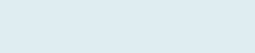# Average Rows with the Same Name

After reading this article you will be able to find average based on the criteria of another column i.e. specific name that is occurring multiple times.

In case you want to calculate the Average based on criteria matched name in a column and multiple rows we can apply this formula.

QUERY

We have columns Customer, Item and Price. Our task is to get the average of the price in multiple rows with specific customers in column (B).

Formula to implement:

=AVERAGEIF(\$B\$3:\$B\$15,B\$4,\$D\$3:\$D\$15)

Function applicable:

AVERAGEIF

The Excel AVERAGEIF Function calculates the average (arithmetic mean) of the numbers in a range that meet the applied criteria. The criteria for AVERAGEIF supports logical operators (>, <, <>, =) and wildcards (*,?) for partial matching.The AVERAGEIF function has the following arguments, the first 2 are required, the last one is optional:

Range: A lookup range to apply criteria.

Criteria: The criteria that must satisfy in the given Range.

Average_ range [Optional]: The range of cells you actually want to average if it satisfies the condition.

Formula Explanation (Step by Step)

You can also debug formula using shortcut F9 function key or under formulas, tab clicks on to evaluate the formula.

Let’s break the formula into multiple small steps to understand its application.

First formula

IF(\$B\$3:\$B\$15,B\$4)

Note: Function AVERAGEIF  is a combination of AVERAGE and IF.

Step 1:Result: 3

It checks in the range (B3 to B15) with criteria name – “preya” and then return how many times given text appear in column – 3 times in our case.

Step 2:

=AVERAGEIF(\$B\$3:\$B\$15,B\$4,\$D\$3:\$D\$15)

Result: 41.67

(50+40+35)= 125

(125/3)= 41.67        ‘Sum next cell value divided by number of occurrence

Now it sums all prices given in the next column matching name i.e. Preya in the given range (D3 to D15) then divide it by counts.

Go ahead and simply change the customer name or price to see how formula works 🙂